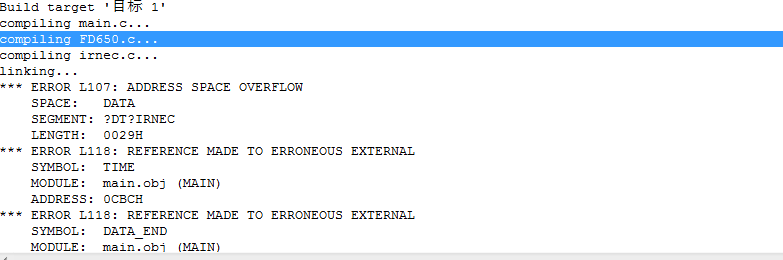```/********************************************************************

*/
#include <fd650.h>
MS_U8 G_num = 0;   //全局数字

int num ;
if(num!=0){
G_num = num;
}
}

void ET_0() interrupt 1 using 1                //定时器0中断
{
}

void EX_0() interrupt 0                //外部中断0,按键按了就中断下
{
LED1 = ~LED1;	  //简单的灯开关
G_num++;

}

void ET0_init()                //定时器0中断初始化
{
TMOD=0x02;                //定时器0工作在方式2
TH0=0x00;                //重载值
TL0=0x00;                //初始值
ET0=1;                //开启定时器0中断
TR0=1;
}

void EX0_init()                //外部中断0初始化
{
IT0=1;                //设置外部中断0为下降沿触发
EX0=1;                //开启外部中断0
EA=1;                //开启总中断
}

void main()
{
int mm = 0;
MDrv_FrontPnl_Update((MS_U8*)" OFF",0);	   //来个初始显示，可以直接显示字符串的，传入文本指针
ET0_init();
EX0_init();
LED1 = 0;
while(1)
{
MDrv_FrontPnl_Update(mystring(G_num), 0);
}
}
}```